Next: Endomorphism Rings over Number Up: Invariants of Modular Abelian Previous: Invariants of Modular Abelian   Contents

# Endomorphism ring of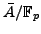Let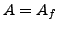be a modular abelian variety over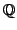associated to a newform in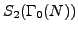. Let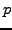be a prime of good reduction for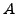(so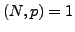). Let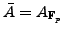be the reduction of themodulo, which is an abelian variety over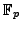.

Problem 8.1.1   Compute the endomorphism ring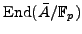.

The endomorphism ring ofcontains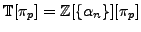, where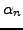is the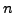-th coefficient of the cusp form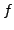of, and the Frobenius endomorphism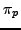satisfies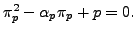If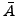is ordinary (i.e. has-rank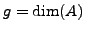), then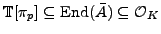where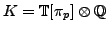and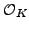is its maximal order. These reductions moduloare CM abelian varieties, but in general only the real subring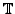generated by the trace terms lift back to the modular abelian variety over.

Note that the invariant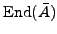is an invariant of the isomorphism class, but not the isogeny class, of. For instance the isogeny class of elliptic curves of conductor 57 denoted 57C by Cremona, consists of two curves: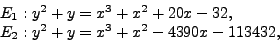such that there exists a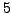-isogeny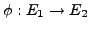between them. This induces isogenies on the reductions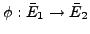, from which one concludes, for each, that eitheris a split or ramified prime in, or thatdivides the index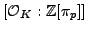, and the two local endomorphism rings differ by index 5: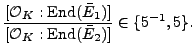If we consider among the first 1000 primes those for which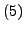is inert in, we can tabulate indices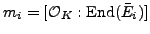: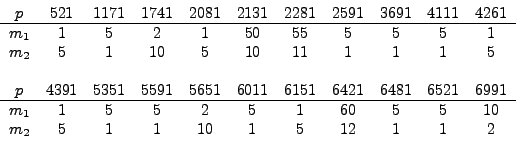The primes for whichis inert inare rare, and that there is no obvious preference for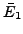or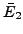to have the larger endomorphism ring. Can one determine a density of primesfor whichis inert in?

Note that the condition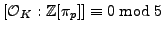is equivalent, up to isomorphism, to the action ofonbeing: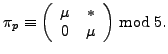The additional condition that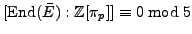is measured by the condition: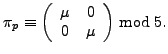Note that there a similar number of primes of supersingular reduction among the first 1000 primes, yet they are known to form a set of density zero.

Problem 8.1.2   Implement in SAGE an algorithm to compute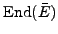for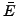an elliptic curve over a finite field. (Does this problem make sense for the special fiber of a Néron model as well?)

For higher dimensional modular abelian varieties, it would be interesting to have algorithms to determine the exact endomorphism rings at, and to characterize the primes at which the reductionhas-rank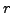in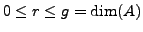.

Problem 8.1.3   Letbe an abelian variety of dimension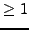attached to a newform and letbe a prime of good reduction. Find an algorithm to compute the exact endomorphism ring.

Problem 8.1.4   Letbe an abelian variety of dimensionattached to a newform. Give an algorithm to compute set of primes at which the reductionhas-rankwith.

Note that the endomorphism rings at ordinary primes are CM orders, and the canonical lift of the reductionis a CM abelian variety. A database of invariants of CM moduli for small genus would aid in classifying these endomorphism rings (at small primes).

Problem 8.1.5   Create a database of invariants of CM moduli for small genus.Next: Endomorphism Rings over Number Up: Invariants of Modular Abelian Previous: Invariants of Modular Abelian   Contents
William Stein 2006-10-20